Question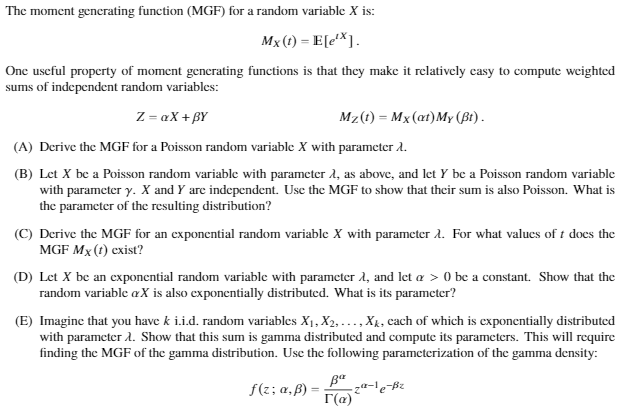The Moment Generating function uniquely defines the distribution of a random variable.

So, for any unknown distribution, we calculate the moment generating function and compare them to a known Moment generating function(of known distributions).

If the calculated Moment generating function of the unknown distribution has the same form as that of the known MGF of some Known distribution, then the unknown distribution has the same probability distribution as that of the known distribution.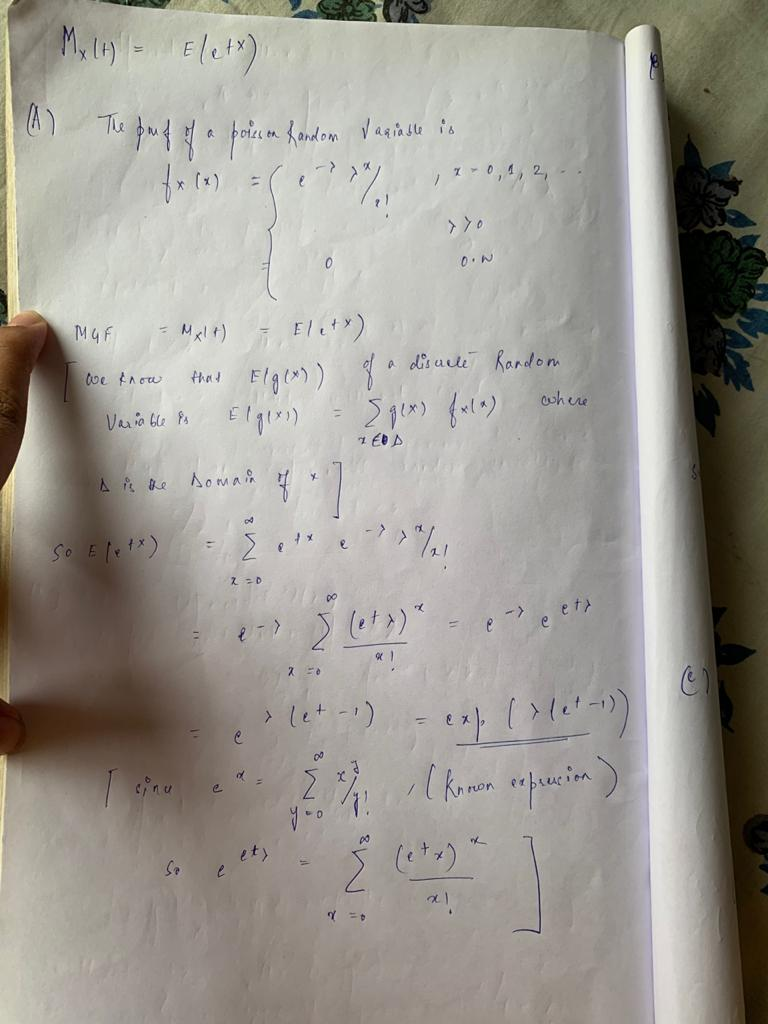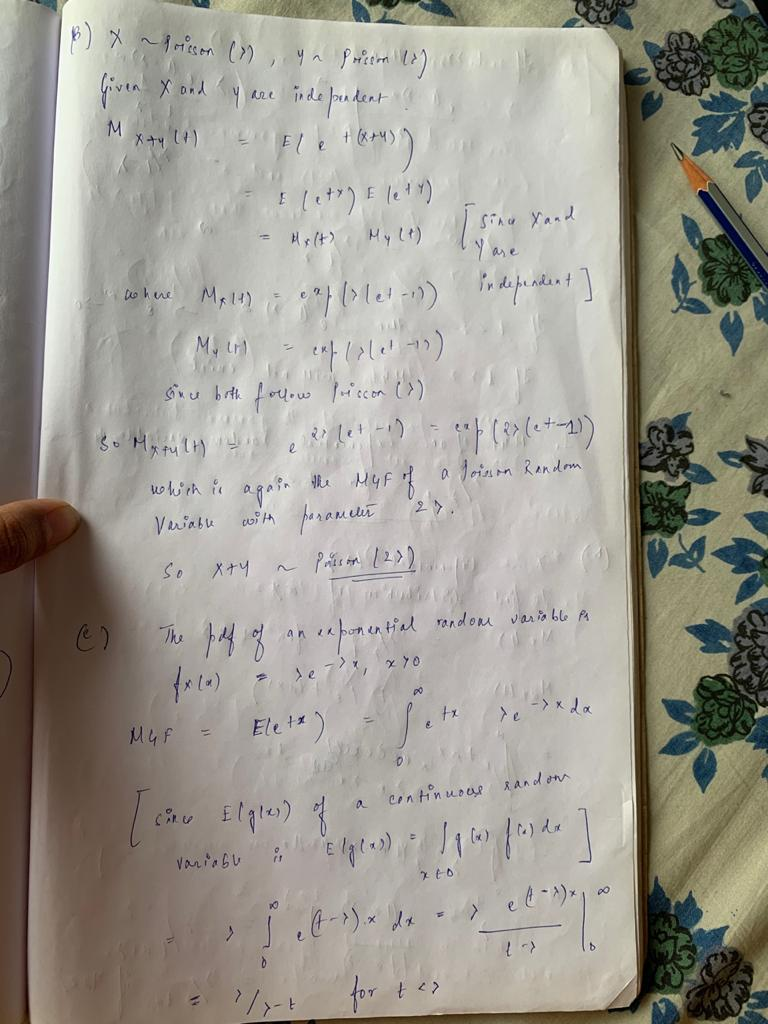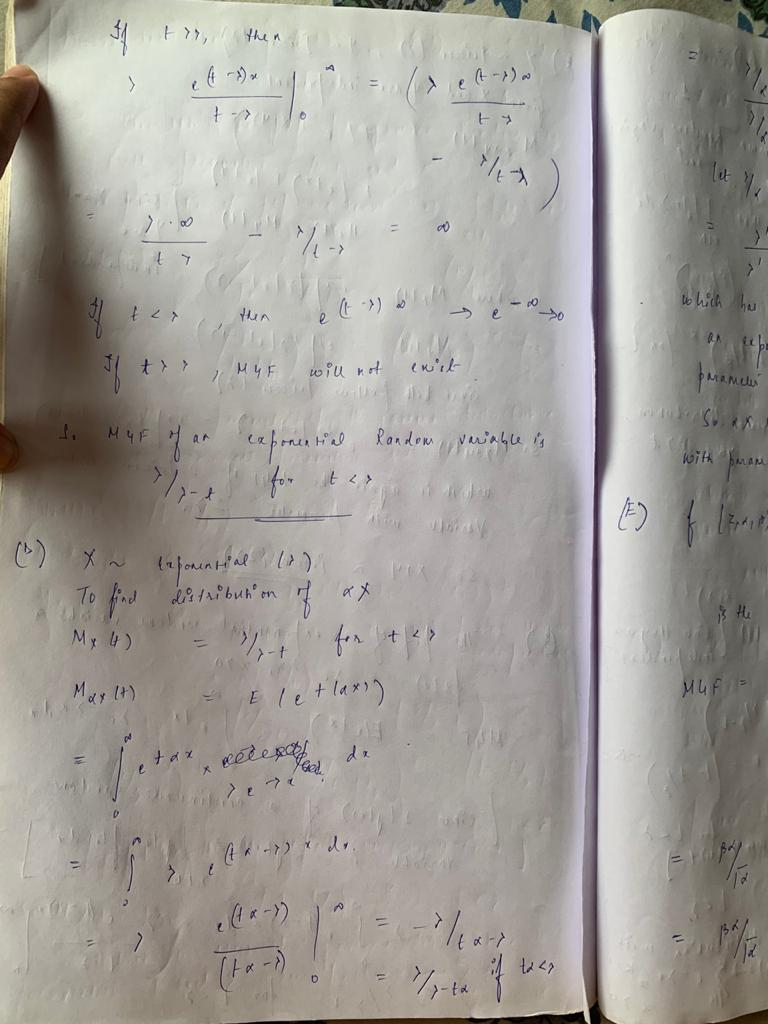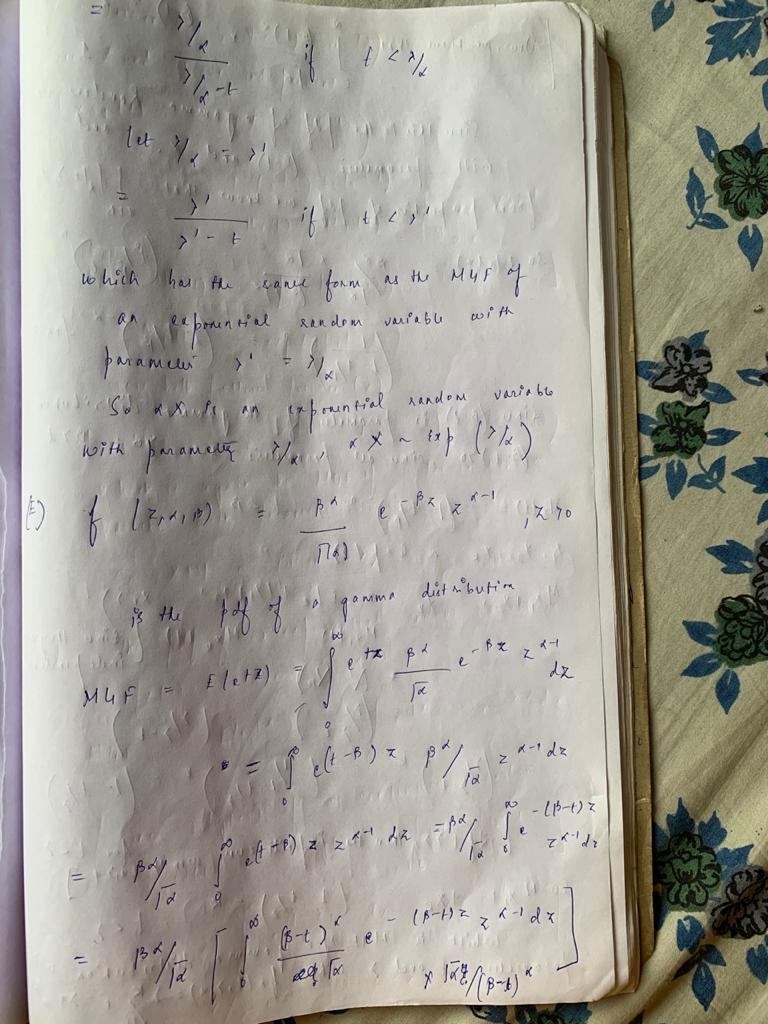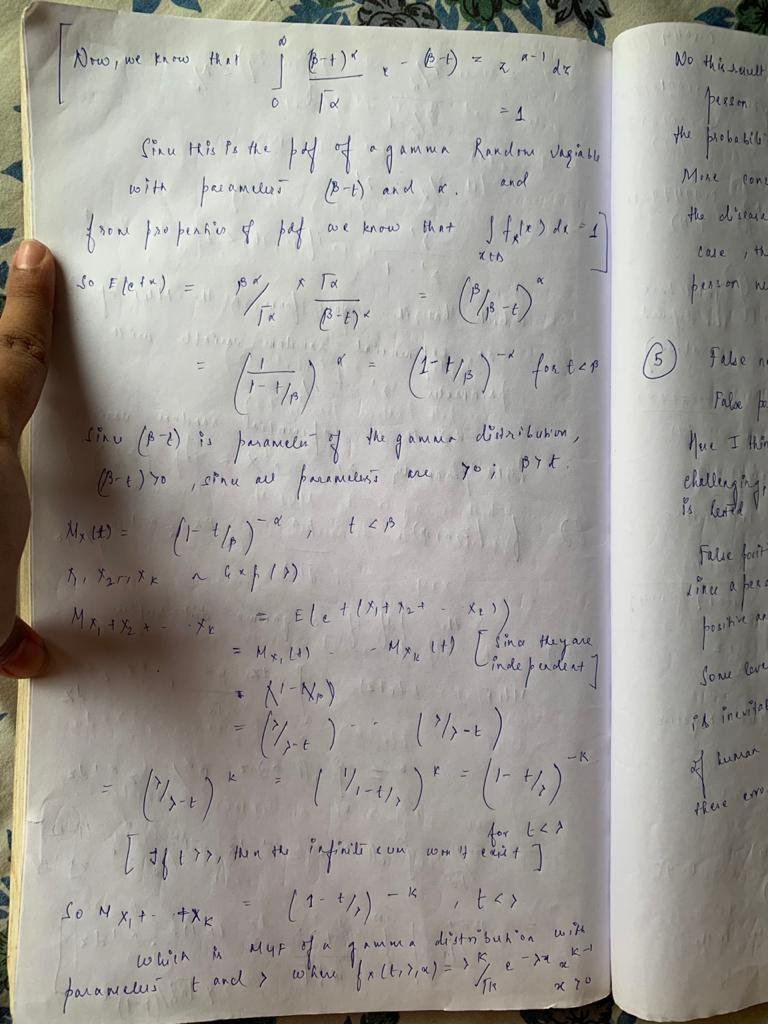#### Earn Coins

Coins can be redeemed for fabulous gifts.

Similar Homework Help Questions
• ### Random variable X has MGF(moment generating function) gX(t) = , t < 1. Then for random...

Random variable X has MGF(moment generating function) gX(t) = , t < 1. Then for random variable Y = aX, some constant a > 0, what is the MGF for Y ? What is the mean and variance for Y ?

• ### 12. let Mx(1) be the moment generating function of X. Show that (a) Mex+o(t) = eMx(at)....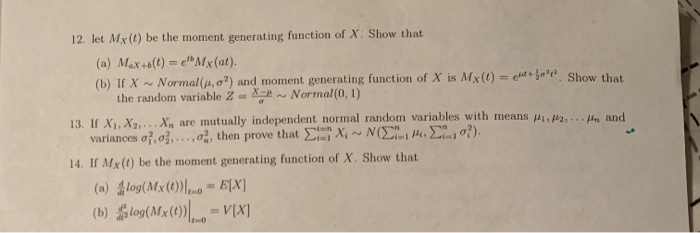12. let Mx(1) be the moment generating function of X. Show that (a) Mex+o(t) = eMx(at). (b) TX - Normal(), o?) and moment generating function of X is Mx (0) - to'p. Show that the random variable 2 - Normal(0,1) 13. IX. X X . are mutually independent normal random variables with means t o ... and variances o, o,...,0, then prove that X NOEL ?). 14. If Mx(1) be the moment generating function of X. Show that (a) log(Mx...

• ### A random variable X has moment generating function (MGF) Problem 1. Mx(s) = (n-0.2 + 0.2e2")2...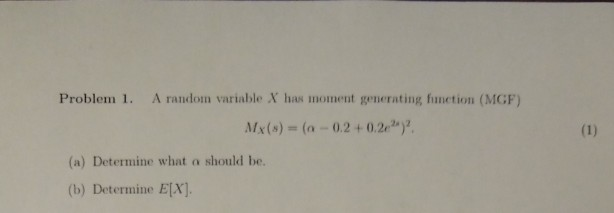A random variable X has moment generating function (MGF) Problem 1. Mx(s) = (n-0.2 + 0.2e2")2 (a) Determine what a should be. (b) Determine E[X].

• ### problem 3 and 4 please. 3. Find the moment generating function of the continuous random variable...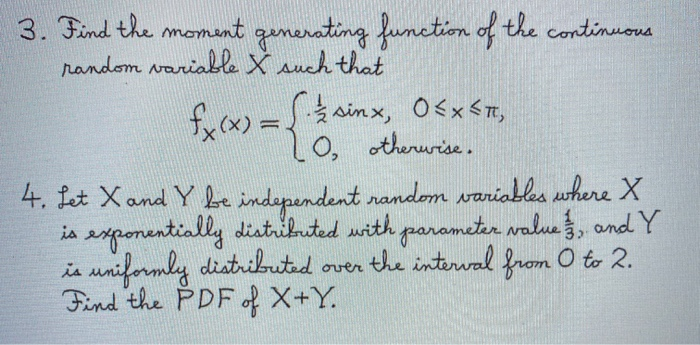problem 3 and 4 please. 3. Find the moment generating function of the continuous random variable & such that i f(x) = { 2 sinx, Ox CT, no otherwise. 4. Let X and Y be independent random variables where X is exponentially distributed with parameter value and Y is uniformly distributed over the interval from 0 to 2. Find the PDF of X+Y.

• ### (1 point) In Unit 3, I claimed that the sum of independent, identically distributed exponential r...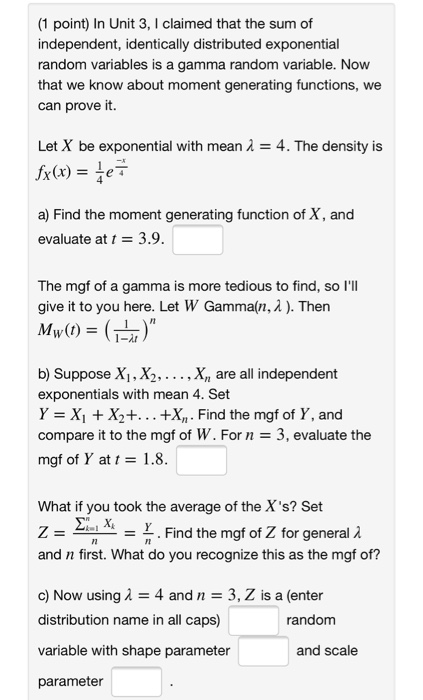(1 point) In Unit 3, I claimed that the sum of independent, identically distributed exponential random variables is a gamma random variable. Now that we know about moment generating functions, we can prove it. Let X be exponential with mean A 4. The density is 4 a) Find the moment generating function of X, and evaluate at t 3.9 The mgf of a gamma is more tedious to find, so l'll give it to you here. Let W Gamma(n, A...

• ### (3 marks) The moment generating function of a random variable X is given by MX(t) =...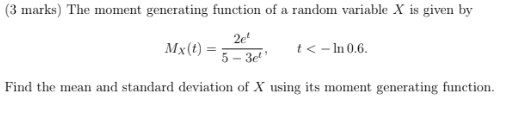(3 marks) The moment generating function of a random variable X is given by MX(t) = 24 20 < - In 0.6. Find the mean and standard deviation of X using its moment generating function.

• ### The moment generating function ф(t) of random variable X is defined for all values of t by et*p(x), if X is discrete e...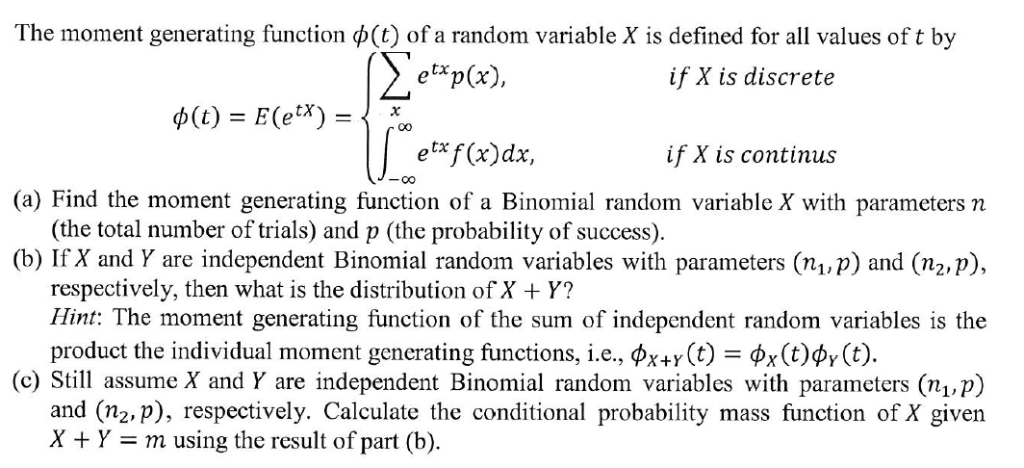The moment generating function ф(t) of random variable X is defined for all values of t by et*p(x), if X is discrete e f (x)dx, if X is continus (a) Find the moment generating function of a Binomial random variable X with parameters n (the total number of trials) and p (the probability of success). (b) If X and Y are independent Binomial random variables with parameters (n1 p) and (n2, p), respectively, then what is the distribution of X...

• ### 6. (4 marks) The moment generating function (mgf) of a random variable X is given by...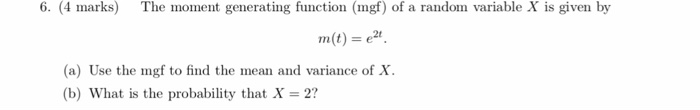6. (4 marks) The moment generating function (mgf) of a random variable X is given by m(t)-e2 (a) Use the mgf to find the mean and variance of X (b) What is the probability that X-2?

• ### (4 marks The moment generating function (mgf) of a random variable X is given by (a)...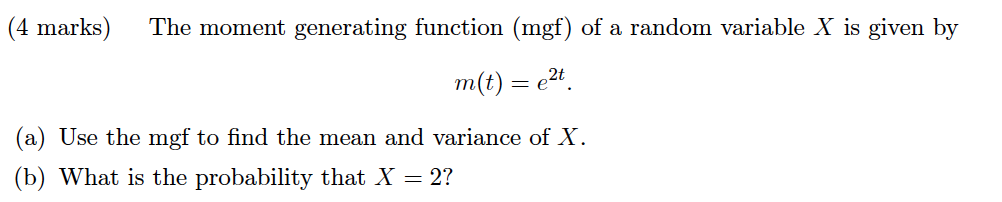(4 marks The moment generating function (mgf) of a random variable X is given by (a) Use the mgf to find the mean and variance of X (b) What is the probability that X = 2?

• ### 3. Let X be a random variable and denote by Mx(t) its MGF. Prove that, for any t > 0, we have 3. Let X be a random variable and denote by Mx(t) its MGF. Prove that, for any t > 0, we have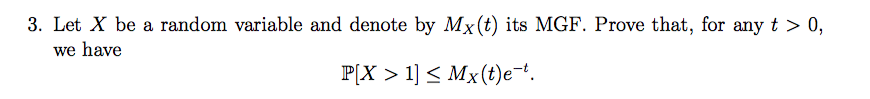3. Let X be a random variable and denote by Mx(t) its MGF. Prove that, for any t > 0, we have 3. Let X be a random variable and denote by Mx(t) its MGF. Prove that, for any t > 0, we have